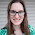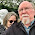## Saturday, July 5, 2014

### #70Days: Strategic Teaching with Compare/Contrast

30/70

One of my favorite units to teach is systems of equations.  For Algebra 2 it is mostly review, although we go deeper into solving 3-variable systems and link it to matrices.  I like the problem solving that goes with systems - much of it is relevant and interesting.

Since students are familiar with linear equations, graphing them, and solving simple systems, I want to use more problem solving to get at characteristics of systems.

In Silver, Harvey F., Richard W. Strong, and Matthew J. Perini. The strategic teacher selecting the right research-based strategy for every lesson. Alexandria, VA: Association for Supervision and Curriculum Development, 2007, one strategy mentioned in chapter 5 is compare/contrast. If you also read Marzano's work, you know that compare/contrast is one of his nine strategies shown to increase student achievement.

I have two problems that I want students to compare. The problems are from Illuminations and Figure This - both works from NCTM. The problems are fun and illustrate some key issues with systems of equations.

I might set up students' work using a matrix discussion guide.  Students will work in pairs (or groups).

Problem 1:  Daniel bought 1 pound of jelly beans and 2 pounds of chocolates for \$2.00. A week later, he bought 4 pounds of caramels and 1 pound of jelly beans, paying \$3.00. The next week, he bought 3 pounds of licorice, 1 pound of jelly beans, and 1 pound of caramels for \$1.50. How much would he have to pay on his next trip to the candy store if he bought 1 pound of each of the 4 kinds of candy?  (http://illuminations.nctm.org/Lesson.aspx?id=2783)

Problem 2: Which cost more - a smile or a frown?

Students will respond to these prompts:

1. What question is asked in each problem?
2. How could you use a drawing to make sense of the problem?
3. What formulas, models or equations could be used?
4. How did you solve the problem?

The first problem doesn’t ask you to solve the system.  And in fact there is not enough information to solve the system.  But you do manipulate linear equations to get to the answer.  The second problem is much simpler.  There are 3 variables, but with close examination there are 2 rows with just 2 variables, so the solution is found fairly easily.

I think it would be interesting to see what skills students use to solve the problems before giving them detailed methods. So I’m planning to use these as an introductory “grit” opportunity before giving notes on solving systems using algebraic methods.

After students have worked on these two problems, we will discuss the similarities and differences.  Questions to consider are:
• Are these two problems more alike or different?
• What is the most important difference?
• What is the effect of the differences?
• What are strategies for solving problems that have multiple variables?

Last, I’ll assign one or both of these problems as homework:
1. Which values should be given to a, b, and c so that the linear system shown has (-1, 2, -3) as its only solution?
x + 2y - 3z = a
-x - y + z = b
2x + 3y - 2z = c

2.  Create your own word problems using the examples today as models.  Explain how they are similar to the problems worked in class.  Illustrate your solutions in detail.

After discussing systems of linear equations we also study systems of inequalities and do a short lesson on linear programming. Do you have an interesting problem to discuss that involves linear optimization? If so, please share!

#### 2 comments:

1.Awesome problems! Thanks for sharing! These make me more excited about teaching systems this year.

1.I had noticed these last year but didn't use them. I'm excited about using them this year as the compare/contrast. I think it will be interesting to see what students do with the candy problem in particular.

I used shape problems similar to the smile/frown problem last year. They are on someone's math blog!

Right now I'm looking for interesting linear programming problems!